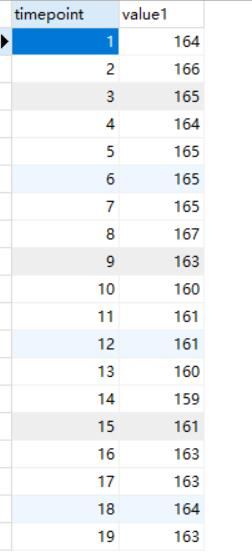``````
<!DOCTYPE html>

<html>

<meta charset="UTF-8">
<title></title>
<!--<script type="text/javascript" src="jquery.js"></script>-->
<script type="text/javascript" src="http://echarts.baidu.com/gallery/vendors/echarts/echarts.min.js"></script>
<script src="http://apps.bdimg.com/libs/jquery/2.1.4/jquery.min.js"></script>
<body>
<div id="main" style="width: 600px;height:400px;"></div>
</body>

</html>
<script>
var app = {
xvalue: [],
yvalue: [],
z:[],
};
// 发送ajax请求，从后台获取json数据
getData();
console.log(app.value1);
console.log(app.timepoint)
console.log(app.predictvalue1)
});

function getData() {
\$.ajax({
url: '/test',
data: {},
type: 'POST',
async: false,
dataType: 'json',
success: function (data) {
app.value1 = data.value1;
app.predictvalue1=data.predictvalue1;
value1 = app.value1;
predictvalue1=app.predictvalue1;
function trueData(i) {
now = new Date(+now + oneDay);
value = value1[i];
return {
name: now.toString(),
value: [
[now.getFullYear(), now.getMonth() + 1, now.getDate()].join('/'),
Math.round(value)
]
}
}
function predictData(i) {
now1 = new Date(+now1 + oneDay);
predictvalue = predictvalue1[i];
return {
name: now1.toString(),
value: [
[now1.getFullYear(), now1.getMonth() + 1, now1.getDate()].join('/'),
Math.round(predictvalue)
]
}
}
var data = [];
var predictdata=[];
var now = +new Date(1997, 9, 3);
var now1 = +new Date(1997, 9, 4);
var oneDay = 24 * 3600 * 1000;
for (var i = 0; i < value1.length; i++) {
data.push(trueData(i));
}
for (var i = 0; i < predictvalue1.length; i++) {
predictdata.push(predictData(i));
}
// 基于准备好的dom，初始化echarts实例
var myChart = echarts.init(document.getElementById('main'));
option = {
title: {
text: '动态数据 + 时间坐标轴'
},
tooltip: {
trigger: 'axis',
formatter: function (params) {
params = params;
var date = new Date(params.name);
return date.getDate() + '/' + (date.getMonth() + 1) + '/' + date.getFullYear() + ' : ' + params.value;
},
axisPointer: {
animation: false
}
},
xAxis: {
type: 'time',
splitLine: {
show: false
}
},
yAxis: {
type: 'value',
boundaryGap: [0, '100%'],
splitLine: {
show: false
}
},
series: [{
name: '真实数据',
type: 'line',
showSymbol: false,
hoverAnimation: false,
data: [],
markLine: {
itemStyle: {
normal: {
borderWidth: 1,
lineStyle: {
type: "dash",
color: 'red',
width: 2
},
show: true,
color: '#4c5336'
}
},
data: [{
yAxis: 900
}]
}
},
{
name: '预测数据',
type: 'line',
showSymbol: false,
hoverAnimation: false,
data: [],
markLine: {
itemStyle: {
normal: {
borderWidth: 1,
lineStyle: {
type: "dash",
color: 'blue',
width: 2
},
show: true,
color: '#4c5336'
}
},
data: [{
yAxis: 900
}]
}
}]
};
// 使用刚指定的配置项和数据显示图表。
myChart.setOption(option);
setInterval(function () {
for (var i = 0; i < 1; i++) {
data.shift();
data.push(trueData(i));
}
for (var i = 0; i < 1; i++) {
predictdata.shift();
predictdata.push(predictData(i));
}
myChart.setOption({
series: [{
data: data
},
{
data: predictdata
}]
});
}, 1000);
}
})
}
</script>
</body>
</html>
``````

``````import MySQLdb
import pymysql
import pandas as pd
import numpy as np
import matplotlib.pyplot as plt
from sklearn.preprocessing import MinMaxScaler
from sklearn.metrics import mean_squared_error
from keras.models import Sequential
from keras.layers import LSTM, Dense, Activation,Dropout
import json
import operator
from functools import reduce
import math
import tensorflow as tf
from keras import initializers
import time

@app.route("/")
def hello():
return render_template('new_file.html')

# /test路由    接收前端的Ajax请求
@app.route('/test', methods=['POST'])
def my_echart():
# 连接数据库
conn = MySQLdb.connect(host='127.0.0.1', port=3306, user='root', passwd='123456', db='test', charset='utf8')
cur = conn.cursor()
sql = 'SELECT timepoint,value1 from timeseries'
cur.execute(sql)
u = cur.fetchall()

timepoint = []
value1 = []

for data in u:
value1.append(data)
timepoint.append(data)
print(value1)
# 转换成json格式
jsonData = {}
jsonData['value1'] = value1
jsonData['timepoint']=timepoint
# json.dumps()用于将dict类型的数据转换成str,因为如果直接将dict类型的数据写入json会报错，因此将数据写入时需要用到此函数
j = json.dumps(jsonData)
cur.close()
conn.close()

# 在浏览器上渲染my_template.html模板（为了查看输出数据)
return (j)

if __name__ == '__main__':
app.run(debug=True,port='5000')
``````3个回答SpringMVC： 前端用ajax方式可以获取session中的数据 直接访问控制器无法正确获取session中的数据?

ajax怎么将后台数据显示在前台页面？

Ajax中的post请求服务器成功后，服务器返回数据，怎么解析到页面上？

ajax查询的动态生成的表格数据如何分页

PHP与html页面的数据交互，用AJAX技术

java前后端分离之后前端怎么展示数据

ECharts怎样用AJAX从数据库中调数据？

String s = new String(" a ") 到底产生几个对象？

Linux面试题（2020最新版）

JVM内存结构和Java内存模型别再傻傻分不清了

loonggg读完需要3分钟速读仅需 1 分钟大家好，我是你们的校长。我之前讲过，这年头，只要肯动脑，肯行动，程序员凭借自己的技术，赚钱的方式还是有很多种的。仅仅靠在公司出卖自己的劳动时...

85后蒋凡：28岁实现财务自由、34岁成为阿里万亿电商帝国双掌门，他的人生底层逻辑是什么？...

MySQL数据库面试题（2020最新版）

HashMap底层实现原理，红黑树，B+树，B树的结构原理 Spring的AOP和IOC是什么？它们常见的使用场景有哪些？Spring事务，事务的属性，传播行为，数据库隔离级别 Spring和SpringMVC，MyBatis以及SpringBoot的注解分别有哪些？SpringMVC的工作原理，SpringBoot框架的优点，MyBatis框架的优点 SpringCould组件有哪些，他们...